﻿ 脉冲式超宽带生命探测雷达时间抖动抑制
«上一篇文章快速检索 高级检索

 雷达学报2015, Vol. 4 Issue (4): 439-444  DOI: 10.12000/JR141150

### 引用本文 [复制中英文]

[复制中文]
Liang Fu-lai, Li Zhao, An Qiang, et al.. Suppression of Time-Domain Jitter of Impulse Radio Ultra-Wide Band Radar[J]. . Journal of Radars, 2015, 4(4): 439-444. DOI: 10.12000/JR14115.
[复制英文]

### 文章历史

School of Biomedical Engineering, Fourth Military Medical University, Xi'an 710000, China
Foundation Items: The National Natural Science Foundation of China (61327805, 61201382)
Abstract: For Impulse Radio Ultra-wide Band (IR-UWB) radar, the time jitter transforms the static clutters to dynamic clutters. Thus, strong residue exists and false alarms form after traditional direct current suppression. The effect of the time-domain jitter on the life detection capacity is analyzed, and then the phenomenon that the relative time delays between the coupling echoes can reflect the time jitter is pointed out. Based on the coupling echo, a method of time jitter suppression is proposed. Experimental data demonstrate that the proposed method can effectively suppress the residue of strong static clutter, and further improve the life-detection capacity.
Key words: IR-UWB (Impulse Radio Ultra-Wide Band) radar     Life detection     Time-domain jitter     Coupling echo
1 引言

IR-UWB生命探测雷达利用人体呼吸体动引起的微弱距离变化实现生命信号探测，要求雷达系统具有良好的时间稳定性，即尽量降低系统的时间抖动。

2 时间抖动对生命探测性能的影响分析

 ${s_{\rm{R}}}(t,\tau ) = \sum\limits_{p = 1}^P {{s_{\rm{T}}}(t)} \otimes {h_p}(t) \otimes \delta \left( {t - {{2{R_p}(t,\tau )} \over {\rm{c}}}} \right) \\ \qquad \qquad + \sum\limits_{q = 1}^Q {{s_{\rm{T}}}(t)} \otimes \delta \left( {t - {{2{R_{sc,q}}} \over c}} \right) \\ \qquad \qquad + \sum\limits_{k = 1}^K {{s_{\rm{T}}}(t)} \otimes \delta \left( {t - {{2{R_{mc,k}} \ \ (t,\tau )} \over {\rm{c}}}} \right)$ (1)

 ${R_p}(t,\tau ) = {b_0}{\rm{ + }}{A_{r,p}}{\rm{sin[2\pi }}{f_{r,p}}(t + \tau )] \\ \qquad \qquad + {A_{hb,p}}\sin [2{\rm{\pi }}{f_{hb,p}}(t + \tau ) + ] + {\rm{res}}(t + \tau )$ (2)

 \eqalign{ & {s_{\rm{R}}}(t,\tau )\!\! =\!\! {s_{\rm{T}}}(t - \!\!\!{t_{\rm{s}}}(\tau )) \!\! \otimes \!\! \left[ {\sum\limits_{p = 1}^P {\delta \left( {t - \!\!\!{{2{R_p}(t,\tau )} \over {\rm{c}}} \!- \!\!\! {t_{\rm{a}}}(\tau )} \right) \!\! \otimes \!\! {h_p}(t)} \! + \!\!\! \sum\limits_{q = 1}^Q {\delta \left( {t\! - \!\!\! {{2{R_{sc,q}}} \over {\rm{c}}}\!\! - \!v{t_{\rm{a}}}(\tau )} \right)} \\ \qquad + \sum\limits_{k = 1}^K {\delta \left( {t\! - \!\!\!{{2{R_{dc,k}}(t,\tau )} \!\! \over {\rm{c}}} \!\!-\!\! {t_{\rm{a}}}(\tau )} \right)} } \right] \!\!\!\! = \!\! {s_{\rm{T}}}(t) \!\otimes \!\left[ {\sum\limits_{p = 1}^P {\delta \left( {t \!- \!\!\! {{2{R_p}(t,\tau )} \!\over {\rm{c}}}\! - \!\!\!{t_{\rm{d}}}(\tau )} \right)} \! \otimes {h_p}(t) \!\!\!\!\!\!\!\!\!\!\! \\ + \sum\limits_{q = 1}^Q {\delta \left( {t - {{2{R_{sc,q}}} \over {\rm{c}}} - {t_{\rm{d}}}(\tau )} \right)} +\!\!\! \sum\limits_{k = 1}^K {\delta \left( {t - {{2{R_{dc,k}}(t,\tau )} \over {\rm{c}}} - {t_{\rm{d}}}(\tau )} \right)} } \right] \cr} (3)

3 基于耦合回波的时间抖动抑制 3.1 基本原理

 ${s_{\rm{R}}}(t,\tau ) \approx {s_{\rm{T}}}(t) \otimes \sum\limits_{q = 1}^Q {\delta \left( {t - {{2{R_{c,q}}} \over {\rm{c}}} - {t_{\rm{d}}}(\tau )} \right)} , \\ \qquad \qquad \, t \in ({t_0},{t_1})$ (4)

 ${s_{\rm{R}}}(n - {t_{\rm{d}}}({m_1}),{m_1}) \approx {s_{\rm{R}}}(n - {t_{\rm{d}}}({m_2}),{m_2}), \\ \qquad \qquad \, \qquad \qquad \quad \, n \in ({N_0},{N_1})$ (5)

 ${S_2}(k) \approx {S_1}(k){e^{jk\theta }}$ (6)

 $\theta = 2{\rm{\pi }}Δf[{t_{\rm{d}}}({m_2}) - {t_{\rm{d}}}({m_1})]$ (7)

${s_1}(n)$和${s_2}(n)$的相关函数$r(n)$为

 \eqalign{ & r(n) = {s_1}(n)\mathop \otimes \limits^{N'} {s_2}( - n) \cr & \;\quad \;\, = {1 \over {N'}}\sum\limits_{k = 0}^{N' - 1} {{S_1}(k)} S_2^{\rm{*}}({k_k}){e^{j{{2{\rm{\pi }}} \over {N'}}kn}} \cr & \;\quad \;\, \approx {1 \over {N'}}\sum\limits_{k = 0}^{N' - 1} {{S_1}(k)} S_1^{\rm{*}}(k){e^{ - jk\theta }}{e^{j{{2{\rm{\pi }}} \over {N'}}kn}} \cr & \;\quad \; = {1 \over {N'}}\sum\limits_{k = 0}^{N' - 1} {{{\left| {{S_1}(k)} \right|}^2}} {e^{j{{2\pi } \over {N'}}k(n - {{N'} \over {2{\rm{\pi }}}}\theta )}} \cr} (8)

 ${\widehat {\vartriangle t}_d} = \frac{{{n_{\max }}}}{{N'\vartriangle f}}$ (9)

3.2 处理流程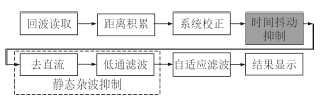图 1 IR-UWB生命探测雷达数据处理流程 Fig.1 Flow chart of IR-UWB life detection radar data processing
3.3 三点讨论

（1）耦合回波的快时间范围选择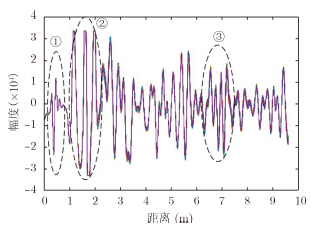图 2 IR-UWB生命探测雷达回波（①内部泄露耦合信号，②收发天线耦合与直达波，③强干扰掩盖下的生命体信号） Fig.2 Echoes of IR-UWB life detection radar (①Coupling signals from internal leak，②Coupling signals between transmitting and receiving antennas and direct wave，③Vital signals shadowed by strong clutters)

（2）参考耦合回波选取

（3）适用性分析

4 试验结果分析图 3 IR-UWB雷达系统实物图 Fig.3 Photo of IR-UWB radar system表 1 IR-UWB雷达系统参数 Tab. 1 Parameters of IR-UWB radar system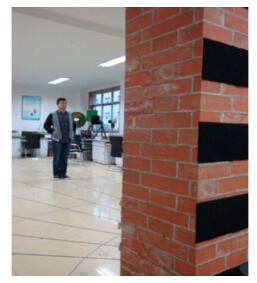图 4 穿墙探测试验场景 Fig.4 Experimental scene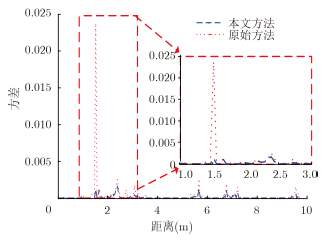图 5 背景数据的处理结果 Fig.5 Processing results of background data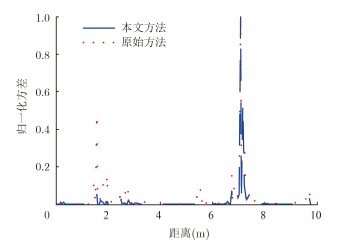图 6 包含单个人体目标的穿墙探测数据处理结果 Fig.6 Results of through wall data including single person
5 结束语

  Li J, Liu L, Zeng Z, et al.. Advanced signal processing for vital sign extraction with applications in UWB radar detection of trapped victims in complex environments[J]. IEEE Journal of Selected Topics in Applied Earth Obersavations and Remote Sensing, 2013, 7(3): 783-791.(1)  Withington P, Fluhler H, and Nag S. Enhancing homeland security with advanced UWB sensors[J]. IEEE Microwave Magazine, 2003, 4(3): 51-58.(1)  Chen K, Zhang J, Norman A, et al.. An X-band microwave life-detection systems for searching human subjects under earthquake rubble or behind barrier[J]. IEEE Transactions on Biomedical Engineering, 2000, 47(1): 105-114.(1)  Barnes M A, Nag S, and Payment T. Covert situational awareness with handheld ultra-wideband short pulse radar[J] , SPIE, 2001, 4374: 66-77.(2)  Nag S and Barnes M. A moving target detection filter for an ultra-wideband radar[C] , Proceedings of the 2003 IEEE Radar Conference, Huntsville, 2003: 147-153.(2)  Xu Y, Dai S, Wu S, et a.. Vital Sign Detection Method Based on Multiple Higher Order Cumulant for Ultrawideband Radar[J]. IEEE Transactions on Geoscience and Remote Sensing, 2012, 50(4): 1254-1265.(1)  谢义方, 方广有. 基于无载频脉冲雷达信号等幅度追踪法检测生命信号[J]. 电子与信息学报. 2009, 31(5): 1132-1135.Xie Yi-fang and Fang Guang-you. Equi-amplitude tracing algorithm based on base-band pulse signal in vital signal detecting[J]. Journal of Electronics & Information Technology, 2009, 31(5):1132-1135.(1)  戴舜, 方广有. 超宽带雷达生命信号频率检测的Cramer-Rao下界[J]. 电子与信息学报. 2011, 33(3): 701-705.Dai Shun and Fang Guang-you. Cramer-Rao lower bound of vital signal frequency detection for ultra wideband radar[J]. Journal of Electronics & Information Technology, 2011, 33(3):701-705.(1)  邵金进, 纪奕才, 方广有,等. 一种用于生命探测雷达的超宽带天线[J]. 电子与信息学报, 2014, 36(2): 471-475.Shao Jin-jin, Ji Yi-cai, Fang Guang-you,et al.. An Ultra-wideband antenna for life detection radar[J]. Journal of Electronics & Information Technology, 2014, 36(2):471-475.(1)  贺峰, 朱国富,牟妙辉,等. 超宽带穿墙雷达对人体动目标探测的实验研究[J]. 现代雷达, 2010, 7(32): 28-33.He Feng, Zhu Guo-fu, Mou Miao-hui, et al.. Experiment study of life locomotion detection based on ultra-wideband through wall radar[J]. Modern Radar, 2010, 7(32):28-33.(1)  Li Y, Jing X, Lü H, et al.. Analysis of characteristics of two close stationary human targets detected by impulse radio UWB radar[J]. Progress In Electromagnetics Research, 2012, 126: 429-447.(1)  Li Z, Li W, Lü H, et al.. A Novel method for respiration-like clutter cancellation in life detection by dual-frequency IR-UWB radar[J]. IEEE Transactions on Microwave Theory and Techniques, 2013, 61(5): 2086-2092.(1)  Xu Y, Wu S, Chen C, et al.. A novel method for automatic detection of trapped victims by ultrawideband radar[J]. IEEE Transactions on Geoscience and Remote Sensing, 2012, 50(8): 3132- 3142.(1)  Rial F I, Lorenzo H, Novo A, et al.. Checking the signal stability in GPR systems and antennas[J]. IEEE Journal of Selected Topics in Applied Earth Observations and Remote Sensing, 2011, 4(4): 785-790.(1)  Liu Q, Wang Y, and Fathy A E. Towards low cost, high speed data sampling module for multifunctional real-time UWB radar [J]. IEEE Transactions on Aerospace and Electronic Systems, 2013, 2(49): 1301-1316.(1)  Wang Y, Zhang Q Y, Yang Z H. A novel method of timing jitter suppression based on UWB system[C]. 2009 International Conference on Wireless Comunications Networking and Mobile Computing, Beijing, 2005:1-5.(1)  张斓子, 陆必应, 周智敏,等. 基于因子分析法和图像对比度的穿墙雷达杂波抑制[J]. 电子与信息学报. 2013, 35(11): 2686-2692.Zhang Lan-zi, Lu Bi-ying, Zhou Zhi-min, et al.. The clutter suppression based on factor analysis and image contrast in through-the-wall application[J]. Journal of Electronics & Information Technology, 2013, 35(11):2686-2692.(1)  Hu J, Zhu G, Jin T, et al.. Study on timing jitter in clutter mitigation of through-wall human indication[C]. 2013 IEEE International Conference on Ultra-Wideband (ICUWB), Sydney, Australia, 2013:211-214.(1)  Li W Z, Li Z, Lü H, et al.. A new method for non-line-of-sight vital sign monitoring based on developed adaptive line enhancer using low centre frequency UWB radar[J]. Progress In Electromagnetics Research, 2013, 133: 535-554.(1)  Lü H, Lu G H, Jing X J, et al.. A new ultra-wideband radar for detecting survivors buried under earthquake rubbles[J]. Microwave and Optical Technology Letters, 2010, 52(11): 2621-2624.(1)